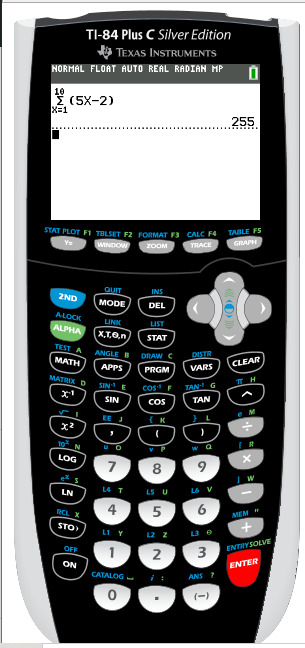# Using Graphic Calculator for AP GP Questions ( Arithmetic and Geometric Progression)In this post, let’s look at the use of graphic calculator for AP GP questions.

You need the graphic calculator to solve some types of questions from the arithmetic and geometric progression chapter. In this post, let’s look at the type of questions where you need functions only available in your graphic calculator.

As we discussed in our earlier post, having a graphic calculator is a must for A Level H2 Math. For each chapter, you will need the graphic calculator to help solve different types of questions. In this post, let’s look at how to use the graphic calculator to solve questions from the arithmetic and geometric progression chapter.

## Graphic Calculator TI-84

The graphic calculator that we will be using for the examples in this post will be the Ti-84 plus graphic calculator, which is approved for use in H2 A Level Math exam at the time of writing this article. This is also the calculator that most junior college uses.Ti-84 Graphic calculator (Source: Amazon.com.)

## Graphic Calculator for AP GP Questions

Well, there are a number of questions from the AP GP chapter where you would help you solve your question faster with a graphic calculator. Honestly, you can solve them manually if you want to, but the use of the GC in these questions will help you check your answer, and speed up the time needed to complete such questions.

The questions from the AP GP topic that needs the graphic calculator are:

• Finding the sum of a given number of terms in an AP or GP
• Finding the nth term for a given condition

## Finding the sum of a given number of terms in the AP or GP progression

The graphic calculator gives answers in numerical values. Hence, if the question is asking for the sum a known number of terms of an AP or GP (or any known number of terms), you can use the GC to do it.

#### Given that the first term of an arithmetic progression is 3, and the common difference is 5, find the sum of the first 10 terms in the AP.

Solution:

rth term of the AP = 3+ (r-1)(5) =5r-2

Using GC,

#### How to type the working from example 1 into the graphic calculator:

In this video below, I show you how to find the first 10 terms of the arithmetic progression (AP) using a Graphic Calculator.

## Finding the nth term for a given condition

Another type of question where you would need the graphic calculator is one where you are asked to find the nth term (usually smallest or largest) when a condition is met (or not met).

Example question 2: Finding the nth term for a given condition to be met

For a GP with first term 5 and common ratio of 1/2, find n such that the sum of the first n terms in the geometric progression exceeds 9.7.

Solution:

rth term of GP =5(1/2)(r-1)

By GC,

Hence, the smallest n value is 6.

#### How to type the working from example 2 into the graphic calculator:

In this video below, I show you what to and how to the working into your graphic calculator to get this:

error: Content is protected !!